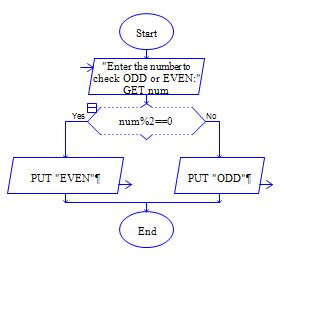# Java Program to find whether the given number is odd or even number

Get a number num and check whether num is odd or even?

Sample Input 1:

45

Sample Output 1:

ODD

Sample Input 2:

56

Sample Output 2:

EVEN

#### Flow Chart Design#### Program or Solution

``` import java.util.*; class OddREve { public static void main(String args[]) { int input1; Scanner sc=new Scanner(System.in); System.out.println("Enter The Input :"); input1=sc.nextInt(); if((input1)%2==0) { System.out.println("The Input is Even."); } else { System.out.println("The Input is Odd."); } } } ```

#### Program Explanation

1. Get input input1 from user using scanner class

2. check whether the remainder of input1 divided by 2 is equal to 0 using if statement.

if it is 0, then

2a. print "input1 is even" using system.out.println.

else

2b. print input1 is odd using system.out.println.